# College Preparatory Mathematics: Help and Review Final Exam

Free Practice Test Instructions:

Choose your answer to the question and click 'Continue' to see how you did. Then click 'Next Question' to answer the next question. When you have completed the free practice test, click 'View Results' to see your results. Good luck!

#### Question 2 2. Find the roots of the equation.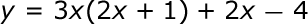#### Question 5 5. Add the following rational expressions.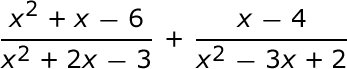#### Question 6 6. Consider the figure below, where Angle 4 = 12m - 4 Angle 5 = 10m + 20 Calculate the measure of Angle 5.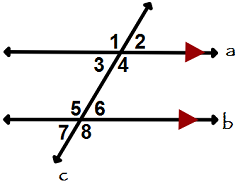#### Question 8 8. Which number is represented by the graph below?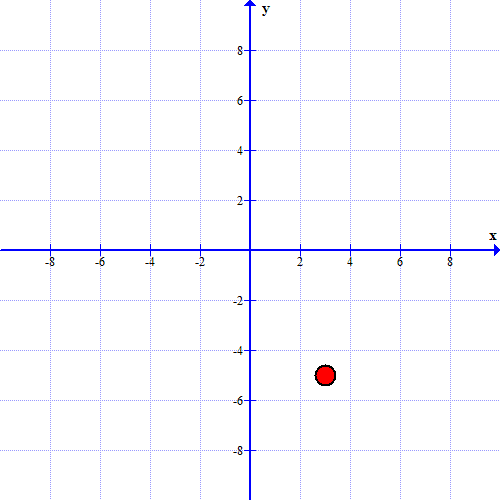#### Question 9 9. Divide the following expressions: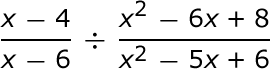#### Question 11 11. Use the Venn Diagram.#### Question 15 15. Given the figure below, Angle 3 = 140y and Angle 5 = 80y + 70. Determine the value of y.